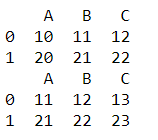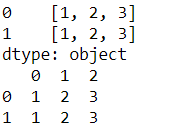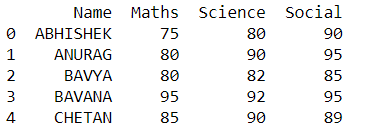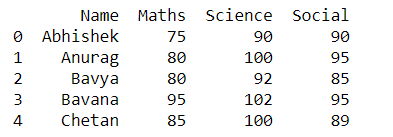Dark Mode On/Off

# Pandas DataFrame apply() Method

In this tutorial, we will learn the python pandas `DataFrame.apply()` method. Using this method we can apply different functions on rows and columns of the DataFrame. The objects passed to the method are Series objects whose index is either the DataFrame’s index (`axis=0`) or the DataFrame’s columns (`axis=1`).

After applying the method, it returns the `Series` or `DataFrame` along the given axis of the DataFrame. This function can be used when we want to alter a particular column without affecting other columns.

The below shows the syntax of the `DataFrame.apply()` method.

### Syntax

``DataFrame.apply(func, axis=0, raw=False, result_type=None, args=(), **kwds)``

### Parameters

func: It represents the function to apply to each column or row.

axis: It represents the axis along which the function is applied, 0 or ‘index’: apply the function to each column, 1 or ‘columns’: apply the function to each row.

result_type: It includes the ‘expand’, ‘reduce’, ‘broadcast’, None, and the default value is None.

These only act when `axis=1` (columns):

• ‘expand’: The list-like results will be turned into columns.

• ‘reduce’: This is the opposite of ‘expand’ and it returns a Series if possible rather than expanding list-like results.

• ‘broadcast’: The results will be broadcast to the original shape of the DataFrame, the original index and columns will be retained.

### Example 1: Applying a `np.sum` function to all the elements of DataFrame using the `DataFrame.apply()` Method

The below example shows how we can apply the function to all the elements of the DataFrame over the axis. Here, in this example, we choose to function as `np.sum`, with `default axis(axis=0)` and `axis=1`.

``````import pandas as pd
import numpy as np
df=pd.DataFrame([[10,11,12],[20,21,22]],columns=['A','B','C'])
print("Applying sum function to all the elements of DataFrame")
print(df.apply(np.sum))
print(df.apply(np.sum,axis=1))``````

Once we run the program we will get the following output.

### Example 2: Applying user defined function to the DataFrame using `DataFrame.apply()` Method

We can pass a user-defined function as a parameter to the `DataFrame.apply()` function. The below example shows the same.

``````import pandas as pd
df=pd.DataFrame([[10,11,12],[20,21,22]],columns=['A','B','C'])
print(df)
return x+1

Once we run the program we will get the following output.### Example 3: Applying lambda function to all the elements of DataFrame using the `DataFrame.apply()` Method

We can pass the lambda function to the `DataFrame.apply() `method. Here, we choose to function as `lambda function`, `axis=1`, `result_type='expand'` and this parameter converts list-like results to columns. The below example shows with and without `result_type` parameter.

``````df=pd.DataFrame([[10,11,12],[20,21,22]],columns=['A','B','C'])
print(df.apply(lambda x: [1,2,3], axis=1))
print(df.apply(lambda x: [1,2,3], axis=1, result_type='expand'))  ``````

Once we run the program we will get the following output.### Example 4: Applying a specific function to a selected column using `DataFrame.apply()` Method

If we want to change a particular column without affecting others, we can use `DataFrame.apply()` method like this. In the below example we are altering only the `'Name'` column by passing the lambda function to the `DataFrame.apply()` method and printing the output.

``````import pandas as pd
df1 = pd.DataFrame([['Abhishek',75,80,90], ['Anurag',80,90,95],['Bavya',80,82,85],['Bavana',95,92,95],['Chetan',85,90,89]], columns=['Name','Maths','Science','Social'])
df1['Name'] = df1['Name'].apply(lambda x: x.upper())
print(df1)``````

Once we run the program we will get the following output.### Example 5: Applying a specific function to a selected column using `DataFrame.apply()` Method

In this example, we are altering the `'Science'` column passing the `lambda function `to the `DataFrame.apply()` method.

``````import pandas as pd
df1 = pd.DataFrame([['Abhishek',75,80,90], ['Anurag',80,90,95],['Bavya',80,82,85],['Bavana',95,92,95],['Chetan',85,90,89]], columns=['Name','Maths','Science','Social'])
df1['Science'] = df1['Science'].apply(lambda x: x+10)
print(df1)``````

Once we run the program we will get the following output.### Conclusion

In this tutorial, we learned the python pandas `DataFrame.apply()` method to the DataFrame. We learned syntax, parameters, and passing different functions, axis, and result types to the `DataFrame.apply()` method, we solved examples. This function is very useful when cleaning up the data like altering particular columns, changing formats, etc.

Want to learn coding?
Try our new interactive courses.
Over 20,000+ students enrolled.